Adapted from a contributed talk at the 2nd Hellenic Cosmology Workshop at NOA (Athens) Jan. 2001

## DARK ENERGY ANTIGRAVITY

### L. Perivolaropoulos

Institute of Nuclear Physics,
National Centre for Scientific Research "Demokritos N.C.S.R.",
Athens, Greece
E-mail: leandros@mail.demokritos.gr

Recent cosmological observations extending the Hubble diagram of high redshift Type Ia supernovae performed independetly by two groups (the Supernova Cosmology Project  and the High-Z Supernova Team [3, 4] presented evidence that the expansion of the Universe is accelerating rather than slowing down. This fact combined with the Boomerang and Maxima-1 measurements of the first acoustic peak location in the angular power spectrum of the cosmic microwave background (CMB) [5, 6] point towards a standard cosmological model with critical density (Ω = Ωm + ΩΛ = 1) and a dominant Λ-like, "dark energy" component at the present epoch (ΩΛ0.7). This component could be produced by non-zero and positive cosmological constant Λ with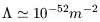(1)

Such a term can produce the required repulsive force to explain the accelerating universe phenomenon. A diverse set of other cosmological observations also compellingly suggest that the universe posesses a nonzero negative pressure component corresponding to vacuum energy density of the same order as the matter energy density [7, 8, 9]

In addition to causing an acceleration to the expansion of the universe the existence of a non-zero cosmological constant would have interesting gravitational effects on various astrophysical scales [10, 1]. For example it would affect gravitational lensing statistics of extragalactic surveys , large scale velocity flows  and there have been some claims that even smaller systems (galactic  and planetary ) could be affected in an observable way by the presence of a cosmological constant consistent with cosmological expectations. Even though some of these claims were falsified [15, 16, 17] the scale dependence of the dynamical effects of vacuum energy remains an interesting open issue.

The effects of the vacuum energy on cosmological scales and on local dynamics can be obtained from the Einstein equations which in the presence of a non-zero cosmological constant are written as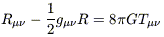(2)

These equations, under the assumptions of spherical symmetric energy-momentum (EM) tensor Tki =Q c2; diag(1, -w, -w, -w) and a mixture of dust-like matter (=m, w = 0) and dark energy (=Q, -1/3w-1) lead (for the 1-1 component) to the generalized Newton's equation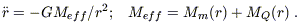(3)

where M(r) = 4π/3 (1 + 3w)r3. Notice that for w < -1/3 we have negative gravitating effective mass (antigravity) which can lead to accelerated cosmological expansion and to non-trivial dynamical effects on astrophysical scales. The accelerated cosmological expansion is obtained for w < -1/3 from the Friedman equations which for k = 0 imply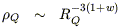(4)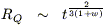(5)

where RQ is the scale factor of the universe. In what follows we focus on the effects of dark energy with w = -1 (cosmological cosntant). The more general case of -1 < w-1/3 () will be discussed elsewhere.

The vacuum energy implied from eq. (1) (10-10 erg/cm3) is less by many orders of magnitude than any sensible estimate based on particle physics. In addition, the matter densitym and and the vacuum energyΛ evolve at different rates, withm /ΛR-3 and it would seem quite unlikely that they would differ today by a factor of order unity. Interesting attempts have been made during the past few years to justify this apparent fine tuning by incorporating evolving scalar fields () or probabilistic arguments based on the anthropic principle ).

For w = -1 we have Einstein's cosmological constant with Λ = 8 π GΛ / c2 (cosmological vacuum with w = -1) and the gravitating mass is MΛ = -8 πΛ r3 / 3. Thus the generalized Newtonian potential leads to a gravitational interaction acceleration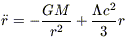(6)

This generalized force includes a repulsive term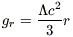(7)

which is expected to dominate at distances larger than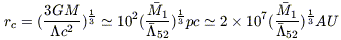(8)

where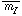1 is the mass within a sphere of radius rc in units of solar masses M= 2 × 1030 kg and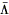52 is the cosmological constant in units of 10-52 m-2.

### REFERENCES

1. M. Axenides, E. G. Floratos and L. Perivolaropoulos, Mod. Phys. Lett. A 15, 1541 (2000) [astro-ph/0004080].
2. S. Perlmutter et al. [Supernova Cosmology Project Collaboration], Ap. J. 517, 565 (1999); astro-ph/9812133.
3. B. P. Schmidt et al. [Hi-Z Supernova Team Collaboration], Astrophys. Journ. 507, 46 (1998); astro-ph/9805200;
4. A. G. Riess et al., Astron. J. 116, 1009 (1998) [astro-ph/9805201].
5. de Bernardis et al. Nature, 404, 955 (2000).
6. Jaffe A.H. et al. astro-ph/0007333 (2000).
7. S. Weinberg, "Theories of the cosmological constant," astro-ph/9610044.
8. L. M. Krauss and M. S. Turner, Gen. Rel. Grav. 27, 1137 (1995) [astro-ph/9504003].
9. S. M. Carroll, W. H. Press and E. L. Turner, Ann. Rev. Astron. Astrophys. 30, 499 (1992).
10. J. P. Ostriker and P. J. Steinhardt, Nature 377, 600 (1995).
11. R. Quast and P. Helbig, Astron. Astrophys. 344, 721 (1999) [astro-ph/9904174].
12. I. Zehavi and A. Dekel, astro-ph/9904221.
13. S. B. Whitehouse and G. V. Kraniotis, astro-ph/9911485.
14. J. Cardona and J. Tejeiro, Ap. J. 493, 52 (1998).
15. E. L. Wright, astro-ph/9805292.
16. I. P. Neupane, gr-qc/9902039.
17. M. D. Roberts, Mon. Not. Roy. Astron. Soc. 228, 401 (1987).
18. R. R. Caldwell, R. Dave and P. J. Steinhardt, Phys. Rev. Lett. 80, 1582 (1998) [astro-ph/9708069]; I. Zlatev, L. Wang and P. J. Steinhardt, Phys. Rev. Lett. 82, 896 (1999) [astro-ph/9807002].
19. J. Garriga and A. Vilenkin, J. Garriga, M. Livio and A. Vilenkin, Phys. Rev. D61, 023503 (2000) [astro-ph/9906210].